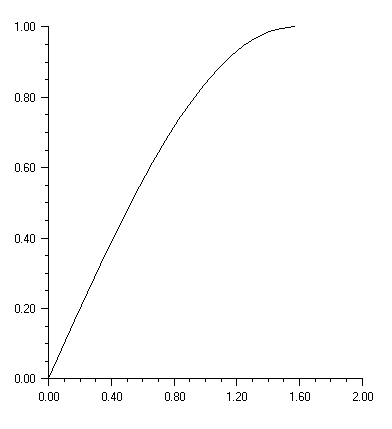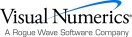# Appendix B: Writing a Chart as a Bitmap Image

An IMSL C# Numerical Libraries chart can be saved as an image file using the .NET Image class.

The chart tree is constructed in the usual manner. Here a method called
CreateChart is used to create a simple chart, with the null-argument Chart constructor. The chart is written to a file without being displayed using the Chart.WritePNG method. This method does not require a desktop GUI application using Windows.Forms, but can be run in a “headless” mode.

If a chart is being displayed, a Bitmap object can be created using the Chart.PaintImage method. This object can then be used like any other .NET Image, including calling its Save method as in this example. For the Image returned by PaintImage not to be null, the chart must have been rendered to the screen. Therefore, this method is not suitable for headless applications.using System;

using System.Drawing;

using System.IO;

using Imsl.Chart2D;

public class SampleImageIO : FrameChart

{

public SampleImageIO()

{

this.Chart = CreateChart();

// Create an image file from the chart's image once displayed

Bitmap bmap = new Bitmap(this.Chart.PaintImage());

bmap.Save("SampleImageIO2.png",

System.Drawing.Imaging.ImageFormat.Png);

}

static Chart CreateChart() {

Chart chart = new Chart();

AxisXY axis = new AxisXY(chart);

int  npoints = 20;

double dx = .5 * Math.PI/(npoints-1);

double[] x = new double[npoints];

double[] y = new double[npoints];

//  Generate some data

for (int i = 0; i < x.Length; i++)

{

x[i] = i * dx;

y[i] = Math.Sin(x[i]);

}

new Data(axis, x, y);

return chart;

}

public static void Main(string[] argv)

{

// Create an image file using WritePNG without a display

Chart chart = CreateChart();

chart.ScreenSize = new Size(500, 500);

FileStream fs =

new FileStream("SampleImageIO.png", FileMode.OpenOrCreate);

chart.WritePNG(fs, 500, 500);

System.Windows.Forms.Application.Run(new SampleImageIO());

}

}Visual Numerics - Developers of IMSL and PV-WAVEhttp://www.vni.com/PHONE: 713.784.3131FAX:713.781.9260